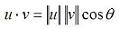# Dot Product (also known as Inner or Scalar Product)

The dot product is a scalar number and so it is also known as the scalar or inner product. In a real vector space, the scalar product between two vectorsis computed in the following way:Besides, there is another way to define the inner product, if you know the angle between the two vectors:We can conclude that if the inner product of two vectors is zero, the vectors are orthogonal.

In Matlab, the appropriate built-in function to determine the inner product is 'dot(u,v)'.

For example, let's say that we have vectors u and v, where

u = [1  0] and v = [2  2]. We can plot them easily with the 'compass' function in Matlab, like this:

x = [1 2]
y = [0 2]
compass(x,y)

x represents the horizontal coordinates for each vector, and y represents their vertical coordinates. The instruction 'compass(x,y)' draws a graph that displays the vectors with components (x, y) as arrows going out from the origin, and in this case it produces:We can see that the angle between the two vectors is 45 degrees; then, we can calculate the scalar product in three different ways (in Matlab code):

a = u * v'
b = norm(u, 2) * norm(v, 2) * cos(pi/4)
c = dot(u, v)

Code that produces these results:
a = 2
b = 2.0000
c = 2

Note that the angle has to be expressed in radians, and that the instruction 'norm(vector, 2)' calculates the Euclidian norm of a vector (there are more types of norms for vectors, but we are not going to discuss them here).

From 'Dot Product' to home

From 'Dot Product' to 'Matlab Examples'

 Top Angle between vectors Cross Product